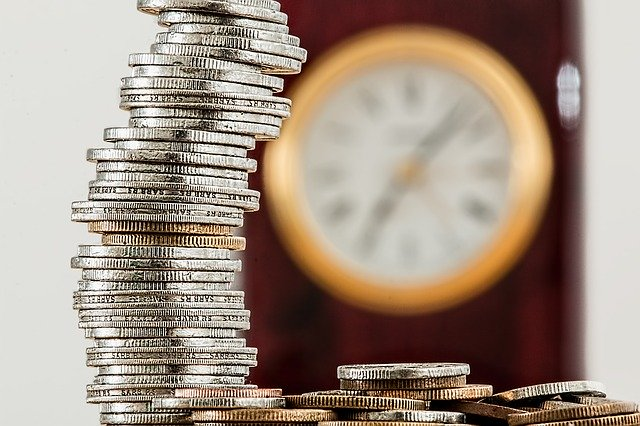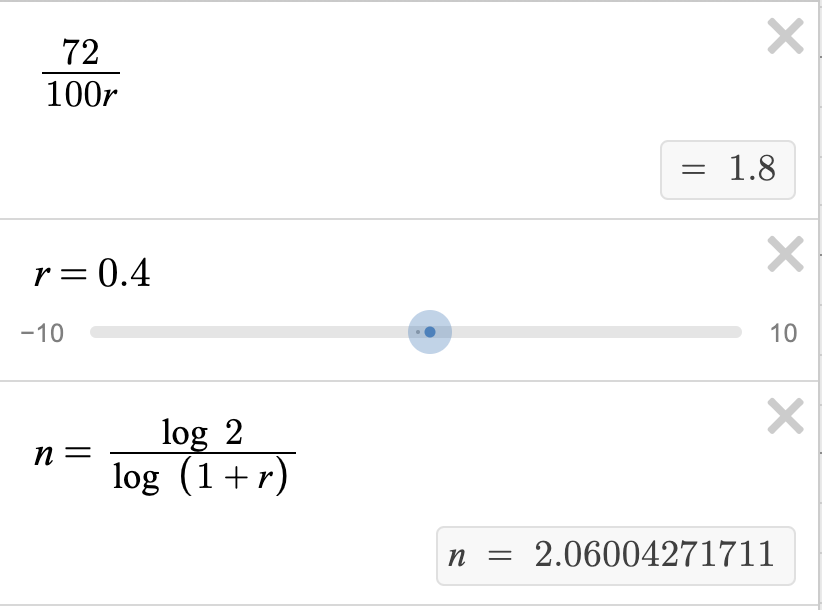## The Rule of 72

The rule of 72 is a handy formula to determine approximately how long an investment will take to double, using a fixed annual rate of interest. It is used for compound interest and is helpful for simple mental approximations.

#### How It Works

All you need to know is the rate of interest. You divide 72 by the interest rate to find how many years it would take to double. For example, we have some money at a 12% interest rate. If we calculate by doing 72/12, we get the answer 6. This means that it takes 6 years for the money to double.

If you are 20 years old, and you put \$1000 into an investment at a 12% interest rate, how much money would you have when you retire at 68 years of age?

Using the previous setup above, we know that our capital will double every 6 years. 72/12 percent interest rate is 6. We will take this money out when we retire, meaning we will have 48 years to grow the money. So, 48 years/6 years to double = 8. This means that our money can double 8 times in 48 years! Just by putting a thousand dollars in an account with good interest rates, we can have our money multiply to become over \$256,000 (\$1,000 x 28), and this isn’t even including additional deposits to the initial principal of \$1000.

Key Takeaway – Even with a slightly higher rate of interest, the time it takes for initial deposit to double is significantly decreased. And given time and interest, our money can multiply before our eyes.

#### What It Tells Us

The rule of 72 is simply a way that investors can calculate how long it takes for our investment to double.

#### The Math Behind Why It Works

To “solve” the rule of 72 equation to understand why it works, we need to look at the formula for compound interest: (P is principal, and 2P is the money doubled)

`2P = P(1+r)n`

Simplifying this equation:

`2 = (1+r)n`

To solve this, we take the log of both sides:

`log 2 = n log (1+r)`

To get n on one side, we divide both sides by log (1+r):

` n = log (2) / log (1+r)`

Now, let’s go back to the rule of 72 itself. Its equation is:

`n = 72 / r`

where “r” is the percent. So let’s turn “r” into “100r”. “100r” is corresponding to the compound interest percentage. So our current rule of 72 equation is:

`72 / 100r`

If we choose a number for “r”, we find that both equations yield a reasonably close calculation for years to double (given r, which is part of the percentage gained).Choosing a number for “r” (we chose 0.4 for 40%) yields us a reasonable estimate of time for capital to double with the rule of 72 (top), relative to the compound interest formula.

#### Conclusion

The rule of 72 tells us in how much time an investment will take to double. Ultimately, it is a useful tool that many investors use as it’s handy and quick in evaluating investment opportunities.